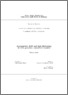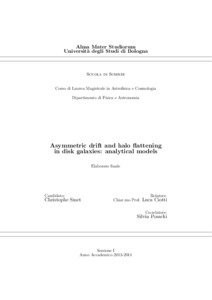# Asymmetric drift and halo flattening in disk galaxies

Smet, Christophe Olivier (2014) Asymmetric drift and halo flattening in disk galaxies. [Laurea magistrale], Università di Bologna, Corso di Studio in Astrofisica e cosmologia [LM-DM270]
Documenti full-text disponibili:Anteprima
Documento PDF

## Abstract

The main result in this work is the solution of the Jeans equations for an axisymmetric galaxy model containing a baryonic component (distributed according to a Miyamoto-Nagai profile) and a dark matter halo (described by the Binney logarithmic potential). The velocity dispersion, azimuthal velocity and some other interesting quantities such as the asymmetric drift are studied, along with the influence of the model parameters on these (observable) quantities. We also give an estimate for the velocity of the radial flow, caused by the asymmetric drift. Other than the mathematical beauty that lies in solving a model analytically, the interest of this kind of results can be mainly found in numerical simulations that study the evolution of gas flows. For example, it is important to know how certain parameters such as the shape (oblate, prolate, spherical) of a dark matter halo, or the flattening of the baryonic matter, or the mass ratio between dark and baryonic matter, have an influence on observable quantities such as the velocity dispersion. In the introductory chapter, we discuss the Jeans equations, which provide information about the velocity dispersion of a system. Next we will consider some dynamical quantities that will be useful in the rest of the work, e.g. the asymmetric drift. In Chapter 2 we discuss in some more detail the family of galaxy models we studied. In Chapter 3 we give the solution of the Jeans equations. Chapter 4 describes and illustrates the behaviour of the velocity dispersion, as a function of the several parameters, along with asymptotic expansions. In Chapter 5 we will investigate the behaviour of certain dynamical quantities for this model. We conclude with a discussion in Chapter 6.

Abstract
Tipologia del documento
Tesi di laurea (Laurea magistrale)
Autore della tesi
Smet, Christophe Olivier
Relatore della tesi
Scuola
Corso di studio
Ordinamento Cds
DM270
Parole chiave
Axisymmetric galaxy models; Jeans equations; analytical solution; asymmetric drift; radial flow.
Data di discussione della Tesi
18 Luglio 2014
URI